Courses

# Quant Mock Test - 2

## 20 Questions MCQ Test Mock Test Series for CLAT 2020 | Quant Mock Test - 2

Description
This mock test of Quant Mock Test - 2 for CLAT helps you for every CLAT entrance exam. This contains 20 Multiple Choice Questions for CLAT Quant Mock Test - 2 (mcq) to study with solutions a complete question bank. The solved questions answers in this Quant Mock Test - 2 quiz give you a good mix of easy questions and tough questions. CLAT students definitely take this Quant Mock Test - 2 exercise for a better result in the exam. You can find other Quant Mock Test - 2 extra questions, long questions & short questions for CLAT on EduRev as well by searching above.
QUESTION: 1

Solution:
QUESTION: 2

### Find the greatest number which divides 285 and 1249, leaving remainder 9 and 7?

Solution:

Clearly required no is 138

QUESTION: 3

### Shopkeeper offers 10% discount on an article and still makes a profit of 20%. What is the CP of the article marked at 500?

Solution:

Marked price = 500
Discount = 10% of 500 = 450
S.P = 450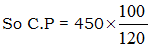= 375

QUESTION: 4

A fair price shopkeeper takes 10% profit on his goods. He lost 20% goods during theft. His loss percent is?

Solution:

Let cost price = 100
Loss during theft = 20
Saleable goods = 80
Profit taken = 10% of 80
= 8
S.P. = 88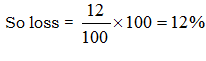QUESTION: 5

If a diagonal of a rhombus are 8 and 6 then the square of its side is?

Solution: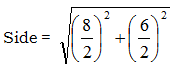= 5
(side)2 = 25

QUESTION: 6

An angle in a semi-circle is?

Solution:
QUESTION: 7

Atyre has 2 punctures, the first can alone empty the tire in 9 min and 2nd can empty the tyre in 6 mins. How long will it take to empty the tyre by both punctures?

Solution: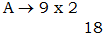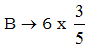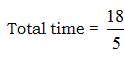QUESTION: 8

In a group of 500 students, 103 students like coco cream and vanilla cream, 300 students like only vanilla cream how students like only coco cream?

Solution: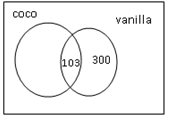So coco only = 500 – 300 – 103  = 97

QUESTION: 9

(764 x?) ÷250= 382

Solution:

(764 x ?) ÷ 250 = 382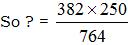= 125

QUESTION: 10

285 is summation of 3 numbers. Ratio between 2nd and 3rd numbers is 6:5. Ratio between 1st and 2nd numbers is 3:7. The 3rd number is?

Solution: It is given that 1:2 = 3:7 and 2:3= 6:5
So 1:2:3=18:42:35
Third no.=35÷95×285
105
QUESTION: 11

A box contains 36 marbles some are blue and others are green. If a marble is drawn at random from a box the probability that it is green is 2/3. Then no. of blue ballsare?

Solution:

No. of blue balls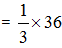= 12

QUESTION: 12

Raman loses 30% of his money after spending 25% of the remaining he still has Rs 525. How much money did he originallyhad?

Solution: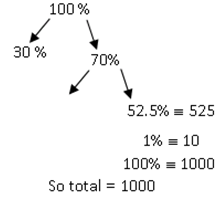QUESTION: 13

If a point (x, y) ina oxy plane is equidistant from (-1, 1) and (4, 3) then?

Solution: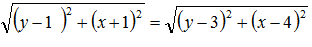x2 + y2 – 2y + 2x + 2 = y2 + x2 + 9 + 16 – 6y – 8x
10x + 4y = 23

QUESTION: 14

15x ? - 8 x 2 – 9 = 0

Solution:

15 x ? – 8 x 2 – 9 = 0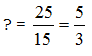QUESTION: 15

If the diameter of a cylinder is 28 cm and its height is 20 cm, then total surface area is?

Solution:

Total surface area = 2πr (r + h)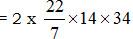= 2992

QUESTION: 16

If the curved surface area of a cylinder is 1760 cm2 and its base radius is 14 cm when it’s height is?

Solution:

Curved surface area = 1760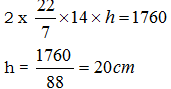QUESTION: 17

Tap A and B can separately fill the tank in 10 hours 15 hours respectively. If both taps are opened together, how much time will it take to fill the tank fully?

Solution: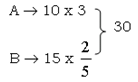So Time taked =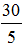= 6 hrs

QUESTION: 18

On selling 100 mangoes, a person gains selling price of 20 mangoes. Find his gain percent?

Solution: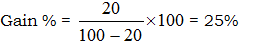QUESTION: 19

The diameter of a wheel is 98cm. Howmuch revolutions will it take to cover a distance of 1540m?

Solution:

Distance covered in one revolution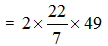= 308 cm
Total revolution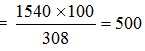QUESTION: 20

Angles ofan quadrilateral are in the ratio 3:4:5:8. The smallest angle is?

Solution:

3x + 4x + 5x + 8x = 360
20x = 360
x = 18
So smallest angles = 18 x 3 = 54°